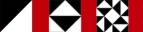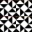Next Higher-Order Parsing 16

# 結合Concatenation

• とりあえずatomの規則はこれひとつだけということにしておこう

Let's pretend for a moment that atom has only this one rule:

```        atom → "FUNC" "(" expression ")"
```
• そうすると、atomはこんな風になる

We could write atom like this:

```        sub atom {
my \$t1 = shift;
my (\$expr, \$t2, \$t3, \$t4, \$t5);

if (   (\$funcname, \$t2) = lookfor("FUNC")->(\$t1)
&& (undef,     \$t3) = lookfor("(")->(\$t2)
&& (\$expr,     \$t4) = expression(\$t3)
&& (undef,     \$t5) = lookfor(")")->(\$t4)) {
my \$val = ... \$funcname ... \$expr ...;
return (\$val, \$t5);
} else {
return;  # failure
}
}
```
• もっとも、ほかの関数もこのパターンになることが多い

But many other functions would also follow this pattern

• だから、小さなパーサをまとめる関数を書くことにしよう

So instead we'll write a function that assembles small parsers into big ones

• A, B,... というパーサ関数があるときに

Given parser functions A, B, etc.:

```        conc(A, B, ...)
```
• とすると A, B, ... と順に探してくれるようにするわけだ

Will return a parser function that looks for A, then B, etc.

 NextCopyright © 2007 M. J. Dominus